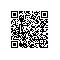# 《从问题到程序：用Python学编程和计算》——3.2　递归

### 3.2　递归

3.2.1　递归定义的函数https://yqfile.alicdn.com/628f75f8e0bb13e9adf1639419cf7cad922e15ea.png" >

def fact(n):
if n == 0:
return 1
else:
return n * fact(n - 1)


def fact(n):
return 1 if n == 0 else n * fact(n - 1)


3.2.2　乘幂的计算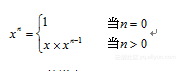def power(x, n):
if n == 0:
return 1
else:
return x * power(x, n-1)


>>> power(2, 1000)
Traceback (most recent call last):
File "<pyshell#0>", line 1, in <module>
power(2, 1000)
... ...https://yqfile.alicdn.com/c6dedcdb3d70e554d90011ea2a4219281729e1b4.png" >

def power(x, n):
if n == 0:
return 1
elif n % 2 != 0:
return x * power(x, n-1)
else:
return power(x*x, n//2)


3.2.3　循环和递归

def power(x, n):
p = 1
while n > 0:
if n % 2 != 0:
p *= x
n -= 1
else:
x *= x
n //= 2
return p


p是这里的累积变量，循环中把x的一些幂次累积到p上，最终得到x的n次幂。循环里区分了几种情况：指数n等于0时累积工作完成，循环结束，变量p的值即为所需。循环体就是一个if语句，两个分支分别处理指数为偶数和奇数的情况，其中用了几个扩展的赋值语句更新变量。注意，对p的初始化保证，即使这个循环的体一次也没有执行，得到的结果也是对的。
3.2.4　斐波那契数列的计算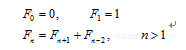def fib(n):
if n < 1:
return 0
if n == 1:
return 1
return fib(n - 1) + fib(n - 2)


0, 1, 1, 2, 3, 5, 8, 13, 21, 34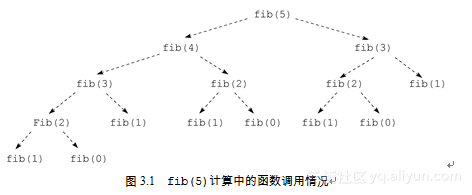• 可能存在一些（许多）从理论上看能用计算机解决的问题，但我们不可能等到计算机给出解的那个时间（人的寿命有限，宇宙的寿命也可能有限）。
• 我们在编程时，需要考虑程序完成计算所需要的时间。计算机科学技术领域最重要的问题之一就是寻找解决各种问题的高效方法（算法）。
• 有些实际问题对于计算完成的时间非常敏感。这方面的例子很多，例如：汽车的ABS/EBD（电子制动分配）系统，必须能在若干分之一秒完成计算；天气预报系统，必须在今天的某个时间完成对明天天气的计算。
对于求Fibonacci数列值的问题，实际上可以找到更好的办法（更好的算法，下面介绍），但有些问题的情况不是这样。人们已经发现了许多非常重要的实际计算问题，从理论上说它们是可以用计算机解决的，不难写出解决它们的程序。但是，从实际角度看这些问题并没有解决，因为对规模稍微大一点的实际情况（“规模大”对应于上面“大一点的参数n”），我们的千万代子孙都无法等到程序给出结果。在这种情况下能说问题解决了吗？显然，说“能”是很牵强的。理解这一点，对于理解计算机也是非常重要的。

Python标准库包time里提供了一个计时函数time，导入这个函数之后，调用它时可以得到一个浮点数，表示从1970年1月1日开始的秒数值。通过两次调用time，就可以为一段计算计时。例如，下面函数可以用于给fib计算计时：

from time import time

def test_fib(n):
t = time()
f = fib(n)
print("Fib[" + str(n) + "] =", f,
"Timing:", str(time() - t) + "s\n")


def test_fibs(m, n):
for k in range(m, n):
test_fib(k)


>>> test_fibs(32, 40)
Fib = 2178309 Timing: 1.4560840129852295s
Fib = 3524578 Timing: 2.1551239490509033s
Fib = 5702887 Timing: 3.5712039470672607s
Fib = 9227465 Timing: 5.6563239097595215s
Fib = 14930352 Timing: 9.236528873443604s
Fib = 24157817 Timing: 14.828848123550415s
Fib = 39088169 Timing: 24.034374952316284s
Fib = 63245986 Timing: 39.42125487327576s


Fibonacci数列的迭代计算

1）F0和F1已知；
2）由F0和F1可以算出F2；
3）一般而言，已知连续两个Fibonacci数，就可以算出序列中下一个数。

def fib(n):
if n < 0:
return 0
f1, f2 = 0, 1 # 开始时分别表示F_0和F_1
k = 0
while k < n:
f1, f2 = f2, f2 + f1
k += 1
return f1

• 首先，进入循环后，第一次判断循环条件时k值为0而f1的值等于0，也就是F0，上述断言关系成立。而且f2的值是F1，是下一个Fibonacci数。
• 假设某次判断循环条件时f1的值是Fk，而且f2的值是Fk+1。循环体里的赋值使f1和f2分别变成Fk+1和Fk+2。随后变量k的值加1，使得f1和f2重新变成Fk和Fk+1。因此，在下次判断循环条件时上述断言仍然成立。
根据上面的论证，我们可以断定函数fib里的循环是正确的，对任何参数n，这个循环都能在算出第n个Fibonacci数后结束。正因为如此，函数fib也是正确的。注意，这一结论具有一般性，与函数的具体参数值无关，因此是一个（数学意义上的）证明。前面说过，一次测试可以说明程序对这一测试实例正确，但多少次能给出百分之百肯定的结论呢？以函数fib为例，其参数可以是任何整数。我们测试了0，结果正确；测试了1，结果也正确；再做一批测试，就能断定对所有的正整数fib都能给出正确结果吗？

ssum = 0
k = 1
while k < 101:
ssum += k * k
k += 1


def fib0(f1, f2, k, n):
if  k > n:
return f1
else:
return fib0(f2, f1 + f2, k + 1, n)

def fib(n):
return fib0(0, 1, 1, n)


def fib(n):
f1, f2 = 0, 1
for k in range(n):
f1, f2 = f2, f2 + f1
return f1


3.2.5　最大公约数

if m % k == 0 and n % k == 0:
d = k;  # k是新的公约数，而且更大


def gcd (m, n):
d = 1
k = 2
while k <= m and k <= n:
if m % k == 0 and n % k == 0:
d = k
k += 1
return d


• 当m和n都是0时不存在最大公约数。上面函数定义没考虑这种情况，给出结果1并不合适。对这种特殊情况需要选一种合理的处理方式，例如可以令函数返回0（0不是任一对整数的最大公约数），表明遇到了特殊情况。
• 如果m、n之一为0，最大公约数就是另一个数。在遇到这种情况时，上面函数返回的值也不对，可以加入直接的判断和处理。
• m、n可能是负数，此时上面函数返回1，一般而言是错的，应该处理。
综合起来，在函数的循环之前应该加上几个语句：
if m == 0:
return n
if n == 0:
return m
if m < 0:
m = -m
if n < 0:
n = -n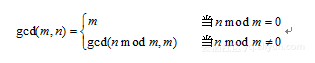def gcd(m, n):
if n % m == 0:
return m
return gcd(n % m, m)


def gcd_main(m, n):
if m < 0:
m = -m
if n < 0:
n = -n
if m == 0:
return n
return gcd(m, n)


1）循环开始时，m和n是求最大公约数的出发点。
2）每次循环判断n % m是否为0。如果为0，m就是最大公约数，结束。
3）否则做变量更新：更新后的n取原来m的值，而m取原来n % m的值。这两个变量更新可以用一个平行赋值实现：

m, n = n % m, m


def gcd(m, n):
if m == 0:
return n
while n % m != 0:
m, n = n % m, m
return m


n的值为0以及m和n的值都为0的情况没有特殊处理，因为这些处理都已经包含在定义里了。读者很容易弄清楚这一点。上面函数里也有一个较复杂的循环。按照前面说法，一个循环中总要维持某种不变关系，以保证循环的正确性。那么，上面函数里的这个循环维持的是变量之间的什么关系呢？这一点也请读者考虑。

3.2.6　不容易用循环求解的递归问题https://yqfile.alicdn.com/d10d577c7fb2085f2f5074380cf3ad122673591d.png" >

1）借助于柱B把63个圆盘从A搬到C；
2）从A搬一个圆盘到B；
3）借助于柱A把63个圆盘从C搬到B。

def henoi(n, sfrom, sto, sby):


def moveone(sfrom, sto):
print("Move a disk from", sfrom, "to", sto)

def henoi(n, sfrom, sto, sby):
if n == 1:
moveone(sfrom, sto)
return
henoi(n - 1, sfrom, sby, sto)
moveone(sfrom, sto)
henoi(n - 1, sby, sto, sfrom)


>>> henoi(3, "A", "B", "C")
Move a disk from A to B
Move a disk from A to C
Move a disk from B to C
Move a disk from A to B
Move a disk from C to A
Move a disk from C to B
Move a disk from A to B


• 用了一个硬币a之后n - a的兑换方式数，加上
• 不用硬币a时n的兑换方式数
这是递归的情况。有几种（基础）情况可以直接得到结果：
• n等于0时，应该认为是找到了一种兑换方式（计1种兑换方式）；
• n小于0，说明没找到兑换方式（计0种兑换方式）；
• 硬币的种类数等于0，说明没找到兑换方式（0种兑换方式）。
由于上面递归的一种方式是去掉一种硬币，因此可能出现最后这种情况。

def amount(k):
if k == 1:
return 1
if k == 2:
return 2
if k == 3:
return 5
if k == 4:
return 10
if k == 5:
return 50
if k == 6:
return 100


def ccoins(k, n):
if n == 0:
return 1
if k == 0 or n < 0:
return 0
return ccoins(k, n - amount(k)) + ccoins(k - 1, n)


def num_coins(n):
return ccoins(6, n)


3.2.7　更复杂的递归情况

def even(n):
if n == 0:
return True
else:
return odd(n-1)


# even(4) # 在这里调用将会报销

def odd(n):
if n == 0:
return False
else:
return even(n-1)